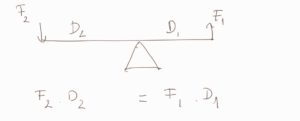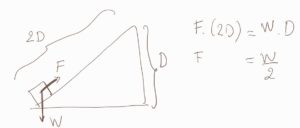Written by Chau Pham on Feb 26, 2020

Mechanical advantage is a measure of the ratio of output force to input force in a system, used to analyze the forces in simple machines like levers and pulleys

Despite changing the forces that are applied the conservation is still true and the output energy is still equal to the input energy. Typically the mechanical advantage is expressed in ideal terms, where there is no losses in energy between the input and output times (no heat, friction loss), also known as 100% efficient systems.The left side is the input energy (or work put into a machine – seesaw), and the right side is the output energy (or work that comes out of a machine, for no-loss energy exchange).

Once you know the ratio of the displacements (d), you can find the relationship between the 2 forces.### KEY TAKEAWAYS

#### Key Points

• We can find the ratio of output force to input force considering Work in equals to Work out.
• Mechanical advantage is expressed in ideal terms, no loss of energy to heat for friction.

#### Key Terms

• Simple machines: a mechanical device that changes the direction or magnitude of a force like wedge, levers, pulleys.

Billing Information